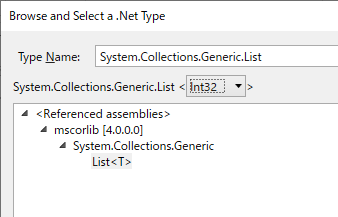# For-loop & assign value to array

Hi

I want to assign value to an array each time, the For-loop iterates. I assign the pValues array variable with the below

pValues = {11*pValues_counter-10}

But pValues becomes a 1x1 array with only the value of the last loop. What is wrong?

Thank you

1 Like

Hi,

There are some ways to achieve it.
I suppose perhaps you should use List as the following image.

Regards,

Hi

Thank you for the reply. Apologies I am unable to upload screenshots or attachments.

Why can’t I use

pValues(pValues_counter) = {11*pValues_counter-10}

pValues_counter is a counter that increase by 1 for each iteration.

Thank you

Hi,

`{ }` means whole the array. So can you try the following expression?

``````pValues(pValues_counter) = 11*pValues_counter-10
``````

Regards,

Hi

Tried

pValues(pValues_counter) = 11*pValues_counter-10

I get the following error:

Source: Assign
Object Reference not set to an instance of an object.

pValues is an array of Int32 and pValues_counter is Int32

Hi,

You need to initialize array before call the assign activity. For example like the following.

``````pValues = New Integer(11){}
``````

Array is for fixed size. If we don’t know its size in advance, need to use List.

Regards,

Hi
How do I select “List” from variable type? When I search “List”, there are many different types of list.
Thank you

Hi,

Can you input `System.Collections.Generic.List` as the following image?Regards,

Hi
How do I assign value to the x index of a list? I keep getting error:

Exception has been thrown by the target of an invocation

My assign is this:

outlier_result(outlier_counter-1) =outlier_name(outlier_counter-1)
outlier_result is the list [of string], and outlier_name is an array of string

All you need is in the screenshot in the first reply from @Yoichi

Working with array is not so convenient as it is fixed size.
The trick @Yoichi used is that instead of array he use a list. With list you could simply add new item to the end of the list (Add To Collection activity).
And finally you convert the list to array (last Assign activity in screenshot)

You should learn a bit if .NET (e.g. here VB.NET List Examples - Dot Net Perls)

Cheers

1 Like